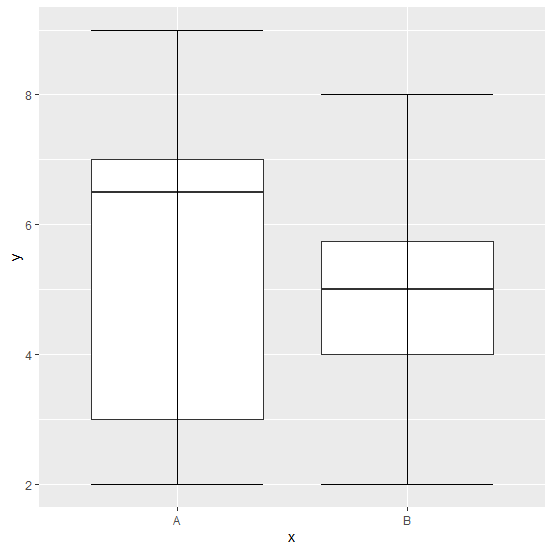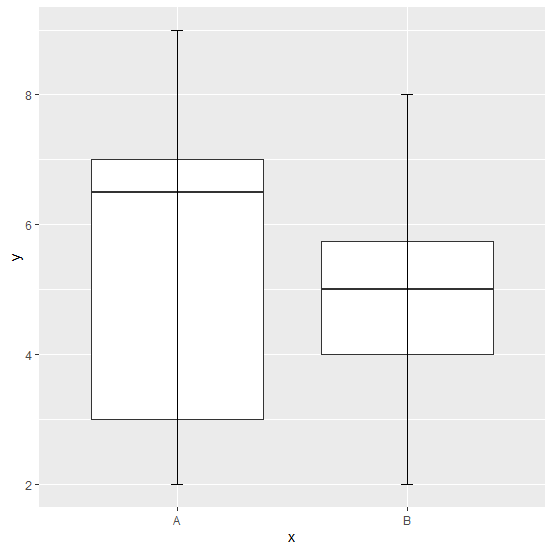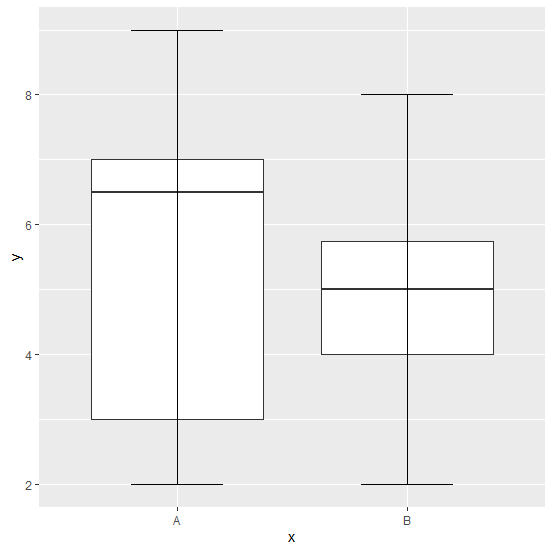# How to change the width of whisker lines in a boxplot using ggplot2 in R?

In R, by default the whisker lines are as wide as the box of the boxplot but it would be great if we reduce that width or increase it because it will get attention of the viewer in that way. This can be done by using the width argument inside the stat_boxplot function of ggplot2 package. Check out the below example to understand how it works.

## Example

Consider the below data frame −

## Example

Live Demo

> x<-sample(LETTERS[1:2],20,replace=TRUE)
> y<-rpois(20,5)
> df<-data.frame(x,y)
> df

## Output

x y
1 B 5
2 B 4
3 A 6
4 A 9
5 B 2
6 B 4
7 B 6
8 B 2
9 B 4
10 B 8
11 B 3
12 A 7
13 B 5
14 A 7
15 A 2
16 B 7
17 B 7
18 A 2
19 B 5
20 B 5

## Example

> library(ggplot2)
> ggplot(df,aes(x,y))+geom_boxplot()+stat_boxplot(geom="errorbar")

## OutputCreating the boxplot with different whisker lines width −

## Example

> ggplot(df,aes(x,y))+geom_boxplot()+stat_boxplot(geom="errorbar",width=0.05)

## Output## Example

> ggplot(df,aes(x,y))+geom_boxplot()+stat_boxplot(geom="errorbar",width=0.40)

## Output